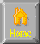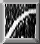```
GAME THEORY
I Zero Sum Games
Solution = mixed strategies from minimax theorem
+ Minimax theorem (1928 J. V. Neumann) Every 2 person zero sum game has a
mixed strategy for each player so expected pay is same value V (optimal strategy, V=best payoff)
+ Zero sum games
R1  R2
S1   2  ->2 row min
S2  1   3  ->1          []=minimax pt.
2   3
col max

+ Optimal Strategies
BI  BII BIII
AI  0  5/6  1/2     vs BI   = 0x+1(1-x)=1-x
AII  1  1/2  3/4     vs BII  = 5/6x + (1-x)/2 = 1/2 + x/3
vs BIII = 1/2x + 3(1-x)/4 = 3/4 - x/4

II NON-COOPERATIVE GAMES
+ Nash's Theorem (1994 John Nash) any n person, noncooperative game (zerosum or nonzero sum)
for which each player has finite number of pure strategies has at least one equilibrium set of strategies

III COOPERATIVE GAMES
+Equilibrium sets. Core of a game, shapley value
+Mixed-Motive Games
Type I - Cuban Missile Crisis      C     D
C (3,3) (2,4)
D (4,2) (1,1)
Type II - Interection Leader       C     D
C (2,2) (3,4)
D (4,3) (1,1)
Type III - Battle of Sexes         C     D
C (2,2) (4,3)
D (3,4) (1,1)
Type IV - Prisoner's Dilemma       C     D
C (3,3) (1,4)
D (4,1) (2,2)

+Multistage cooperative games
+Evolutionary Stable Strategy (ESS)
R. Axelrod - prisoner dilemma games played indefinitely
Tit for Tat - cooperate first ,then do what opponent did previous round
All D
+Emergence of Cooperation
How does non all-D strategy evolve in All D Environment
1. Kin selection - helping one's relatives
2. Clustering - Viability of a small minority employing same strategy

```HomeNext ... Topology